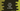# Java program to convert byte to string## Java program to convert byte to string:

In this post, we will learn how to convert byte to string in Java. Byte types can be converted to string in many ways in Java. I will show you a couple of ways in this post with examples.

### Method 1: By using + :

This is the easiest method. We can use + to append the byte value to an empty string to get the string representation of the *byte *value.

Let’s take a look at the below program:

``````class Example{
public static void main(String[] args) {
byte byteValue = 61;

String strValue = byteValue + "";

System.out.println(strValue);
}
}``````

Here,

• We create the byte variable byteValue with 61
• The string conversion is stored in the variable strValue
• The last line is printing the string value

### Method 2: By using Byte.toString():

Byte class provides one toString method which is defined as below:

``public static String toString(byte b)``

This method takes a byte value as the parameter and returns the string representation of the byte value.  If we write the above program using Byte.toString, it will look like as below:

``````class Example{
public static void main(String[] args) {
byte byteValue = 61;

String strValue = Byte.toString(byteValue);

System.out.println(strValue);
}
}``````

You will get similar output with this program.

You can use any of these methods. Byte.toString is recommended because string concatenation is expensive and it creates intermediate strings.